# Forward modelling of circular loop source and calculation of whole area apparent resistivity based on TEM

Forward modelling of circular loop source and calculation of whole area apparent resistivity based on TEM

Jun Zhang YunboLiBaixiangLiu Yanqing Wu Hongchun Yi

School of Geosciences and Info-Physics, Central South University, Changsha 410083, China

CCTE Chongqing Research Institute, Chongqing 400037, China

Key Laboratory of Metallogenic Prediction of Nonferrous Metals and Geological Environment Monitoring (Central South University), Ministry of Education, Changsha 410083, China

Corresponding Author Email:
lyb0112@163.com
Page:
183-198
|
DOI:
https://doi.org/10.3166/TS.35.183-198
| |
Published:
31 August 2018
| Citation

OPEN ACCESS

Abstract:

Based on the basic theory of transient electromagnetic method (TEM) and the exciting field of circular loop sources, this paper derives the calculation formulas for the vertical magnetic field and the induced electromotive force of the circular loop source over layered medium, uses the vertical magnetic field and the kernel function to calculate the whole area apparent resistivity and establishes typical two-layer and three-layer geo-electric models by changing the resistivity and thickness of the layered medium. Then it plots the whole area apparent resistivity curves of different geo-electric models and analyzes their electrical response characteristics. According to the model calculation results, this calculation method is correct and effective, and the smaller the resistivity is, the greater the thickness of the layered medium will be and the larger the response amplitude will be; the calculated whole area apparent resistivity is highly accurate and can truly and objectively reflect the electrical properties of the geo-electric section. Considering that the circular area is the largest with the same perimeter, a circular loop device can increase the emission magnetic moment with the same emission current. Therefore, this method can provide a solid theoretical basis for improving the mine TEM coils and the detection accuracy.

Keywords:

circular loop source, forward modelling, whole area apparent resistivity, geo-electric model, numerical calculation, electrical characteristic response

1. Introduction

The transient electromagnetic method (TEM) is a geophysical method that detects the electrical properties of a medium by transmitting a pulsed electromagnetic field to the ground using an ungrounded loop and observing the variation pattern of the secondary eddy current field with time. The TEM forward modelling is the basis for the theoretical research and the processing and interpretation of measured data. It usually solves the harmonic electromagnetic field in the frequency domain first, and then transforms to the time domain to obtain the transient response through the Fourier transform (Christiansen et al., 2015; Fullagar et al., 2015; Yu et al., 2013; Amaya et al., 2018). The frequency domain response is often solved through the Hankel transform (Anderson, 2012; Guptasarma and Singh, 1997), while from the frequency domain to the time domain, the G-S transform and the sine and cosine transform methods are often used (Wang et al., 1994; Schamper et al., 2014).

The measured transient electromagnetic response curve cannot directly reflect the characteristics of the formation, so the apparent resistivity is often used to process and interpret the measured data. The apparent resistivity is obtained from the field quantities collected and through approximate or non-approximate calculations. The early- or late-time apparent resistivity is just obtained through approximate calculation, and there will be deviations to the calculated result if the early or late conditions are not met. The apparent resistivity obtained through non-approximate calculation is called the whole area apparent resistivity, which is more accurate than the calculation result of the early- or late-time apparent resistivity formula. In recent years, many scholars have conducted in-depth research on the calculation of the whole area apparent resistivity. Bai et al. (2003) proposed a numerical calculation method for the all-time apparent resistivity based on the central-loop TEM; Li et al. (2007) synthesized the loop source by superimposing the horizontal electric dipoles and calculated the whole area apparent resistivity of the arbitrary shape loop source; Wang (2008) used the translation algorithm to calculate the whole area apparent resistivity for the central-loop TEM; Chen (2009) used the binary search method to realize the fast calculation of the transient electromagnetic whole area apparent resistivity; Guo and Wang (2010) calculated the transient electromagnetic whole area apparent resistivity based on the magnetic field and the induced electromotive force.

Based on the previous research results, this paper establishes typical layered medium geo-electric models for forward modelling simulation, and introduces the kernel function to calculate the whole area apparent resistivity. Through the numerical calculation of multiple geo-electric models, this paper verifies the calculation method is correct and accurate, and that the result can truly and objectively reflect the electrical properties of the geo-electric section. Considering that the circular area is the largest with the same perimeter, a circular loop device can increase the emission magnetic moment with the same emission current. Therefore, this method can provide a solid theoretical basis for improving the mine TEM coils and the detection accuracy.

2. Transient electromagnetic field excited by the circular loop source over the layered medium

## 1.jpg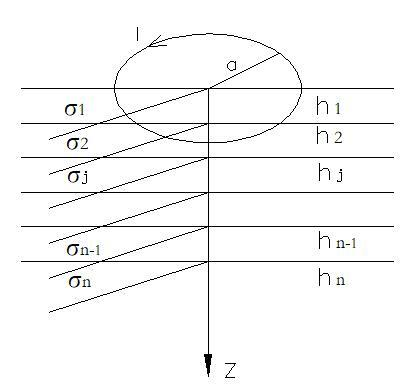Figure 1. Schematic diagram of the emission loop for layered medium

Suppose there is a circular loop over the isotopic horizontal n-layered medium, with a radius of . Let the harmonic current $I=I_{0} e^{i w t}$ flow in the circular loop, and introduce the cylindrical coordinate system, where the origin is the centre of the circular current loop, and the z-axis is vertically downward. The conductivity $\sigma_{j}$ and the thickness $h_{j}$ of each layer are shown in Figure 1. So the vertical magnetic field in the frequency domain is obtained as follows:

$H_{z}(w)=I_{0} a \int_{0}^{\infty} \frac{Z^{(1)} \lambda}{Z^{(1)}+Z_{0}} J_{1}(\lambda a) J_{0}(\lambda r) d \lambda$ (1)

where, Z0 is the input wave impedance of the surface medium; and Z(1) is the input wave impedance in the first layer of medium. The recursion formula is as follows:

\left\{\begin{aligned} Z_{j}=-i w \mu_{0} / u_{j}, & u_{j}=\sqrt{\lambda^{2}+k_{j}^{2}}, k_{j}^{2}=-i w \sigma_{j} \mu_{0} ? \\ Z^{(j)}=Z_{j} & \frac{Z^{(j+1)}+Z_{j} \operatorname{th}\left(u_{j} h_{j}\right)}{Z_{j}+Z^{(j+1)} \operatorname{th}\left(u_{j} h_{j}\right)} \\ Z^{(n)}=Z_{n} \end{aligned}\right. (2)

where, Zj is the input wave impedance of the j–th medium surface; Z(j) is the input wave impedance in the -th layer of medium;  is the wave number of the j-th layer of medium, j=1, 2, ... n-1; and  is the magnetic permeability in vacuum.

When the receiving occurs at the centre of the emission loop, r=0. Since $J_{0}(0)=1$, we have:

$H_{z}(w)=I_{0} a \int_{0}^{\infty} \frac{\lambda Z^{(1)}}{Z^{(1)}+Z_{0}} J_{1}(\lambda a) d \lambda$   (3)

By Transform the harmonic electromagnetic field in the frequency domain into the time domain by the Fourier transform (Maclennan and Li, 2011), and we have the transient response under the step current excitation:

$\left\{\begin{array}{l}{H_{z}(t)=\frac{2}{\pi} \int_{0}^{\infty} \operatorname{Im}\left[I_{0} a \int_{0}^{\infty} \frac{\lambda Z^{(1)}}{Z^{(1)}+Z_{0}} J_{1}(\lambda a) d \lambda\right] \frac{\cos w t}{w} d w} \\ {\frac{\partial H_{z}(t)}{\partial t}=\frac{2}{\pi} \int_{0}^{\infty} \operatorname{Re}\left[I_{0} a \int_{0}^{\infty} \frac{\lambda Z^{(1)}}{Z^{(1)}+Z_{0}} J_{1}(\lambda a) d \lambda\right] \cos w t d w}\end{array}\right.$             (4)

3. Numerical calculation of the TEM field

Equation (4) consists of two layers of integrals, among which, the inner-layer integral is the Bessel function integral, and the outer layer one is the cosine function integral. In this paper, the former is calculated using the Hank transform, and the latter using the G-S transform. And we have (Li et al., 2011):

$\left\{\begin{array}{c}{H_{z}(w)=I_{0} \sum_{m=1}^{140} \frac{Z^{(1)} \lambda_{m}}{Z^{(1)}+Z_{0}} W_{m}} \\ {H_{z}(n, c)=I_{0} c \sum_{n=1}^{12}\left[\sum_{m=1}^{140} \frac{\lambda_{m} Z^{(1)}}{Z^{(1)}+Z_{0}} W_{m}\right] W_{n}}\end{array}\right.$       (5)

$c=\frac{\ln 2}{t}$ is the sampling interval;Wm is the filter coefficient of the Hankel transform (140-point operators); and Wn is the filter coefficient of the G-S transform (12-point operators).

4. Calculation of the whole area apparent resistivity

In the homogeneous half space, the vertical magnetic field and the induced electromotive force at the centre of the horizontal circular loop source are (Fu et al., 2008):

$\left\{\begin{array}{c}{H_{z}(\rho, t)=\frac{I_{0}}{2 a}\left[\frac{3}{\sqrt{\pi} u} e^{-u^{2}}+\left(1-\frac{3}{2 u^{2}}\right) \operatorname{erf}(u)\right]} \\ {\varepsilon(\rho, t)=\frac{\mu_{0} s I_{0}}{4 a t} \frac{1}{u^{2}}\left[3 \operatorname{erf}(u)-\frac{2}{\sqrt{\pi}} e^{-u^{2}} u\left(3+2 u^{2}\right)\right]}\end{array}\right.$    (6)

where, $\operatorname{erf}(u)=\frac{2}{\sqrt{\pi}} \int_{0}^{u} e^{-x^{2}} d x, u=\frac{a}{2} \sqrt{\frac{\mu_{0}}{\rho t}}$ and $\mu_{0}$ is the l vacuum permeability.

The basic idea to solve the whole area apparent resistivity is as follows (Yang et al., 2000 ) under the given emission conditions, the massured value of $H_{z}$ or $\varepsilon$ can $e$ th be obtained, and thus the values of the $H_{z}$ and $\varepsilon$ kernel functions can also be obtained:

\left\{\begin{aligned} z(u) &=\frac{3}{\sqrt{\pi} u} e^{-u^{2}}+\left(1-\frac{3}{2 u^{2}}\right) \operatorname{erf}(u) \\ f(u) &=\frac{1}{u^{2}}\left[3 \operatorname{erf}(u)-\frac{2}{\sqrt{\pi}} e^{-u^{2}} u\left(3+2 u^{2}\right)\right] \end{aligned}\right.   (7)

Given the implicit function relationship between the kernel function z(u), f(u) and u, the value of u can be obtained through searching, although its expression cannot be directly acquired. Finally from the formula $u=\frac{a}{2} \sqrt{\frac{\mu_{0}}{\rho t}}$, the value of $\rho(t)$ can be obtained. This solving process of apparent resistivity does not involve the early-time or late-time approximation, so it is called the whole area apparent resistivity.

## 2.jpg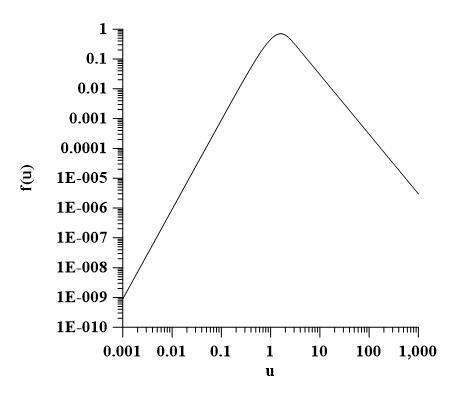Figure 2. Correspondence between the $\varepsilon$ kernel function $f(u)$ and $u$

## 3.jpg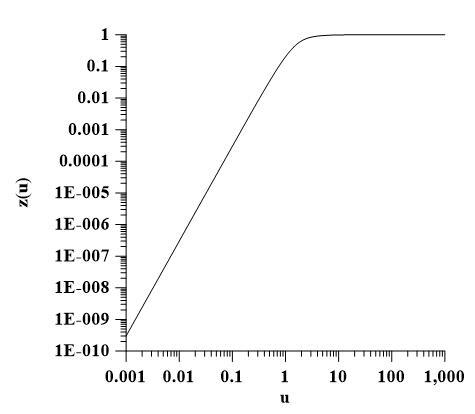Figure 3. Correspondence between $H_{z}$ kernel function $f(u)$ and $u$

As the kernel function f(u) of the induced electromotive force is not a single-valued function of u (as shown in Figure 2), there may be multiple solutions or even no solution for the whole area apparent resistivity defined based on the induced electromotive force. On the other hand, the kernel function z(u) of the vertical magnetic field is a single-valued function of u (as shown in Figure 3), so it only has one unique solution in the whole area. Therefore, this paper calculates the whole area apparent resistivity based on the vertical magnetic field. In the actual engineering detection, the measured induced electromotive force can be converted into the magnetic field through integration (Chen and Tian, 1999; Cui et al., 2015), and then the whole area apparent resistivity can be obtained based on the magnetic field.

5. Model calculation

A geo-electric model of three-layered medium is established, with the resistivity of each layer being 100$\Omega \cdot m$, and the thickness being 50m, 50m and $\infty \mathrm{m}$, respectively. Now we calculate the vertical magnetic field and the whole area apparent resistivity. If we compare the numerically calculated vertical magnetic field of the layered medium with the analytic solution of the magnetic field of the uniform earth with a resistivity of 100$\Omega \cdot m$, we will find the relative error is less than 1% (shown in Figure 4). If we compare the whole area apparent resistivity defined based on the vertical magnetic field with the resistivity set by the model, we will find the relative error is less than 6‰ (shown in Figure 5). This shows that the numerical calculation methods for the vertical magnetic field and the whole area apparent resistivity are correct.

## 4.jpg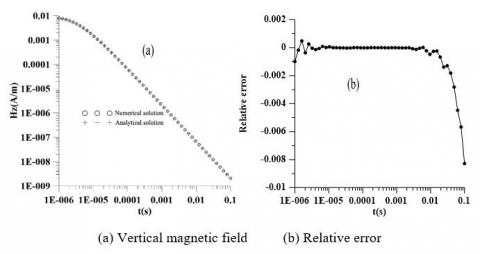Figure 4. Comparison of the numerical result of the vertical magnetic field with the analytical solution and the relative error curve

## 5.jpg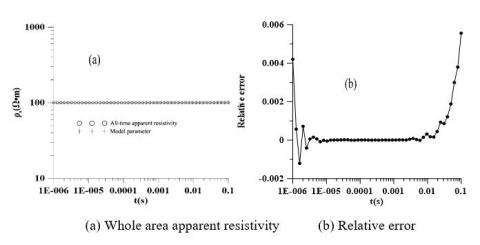Figure 5. Comparison of the numerical calculation result of the whole area apparent resistivity with the model parameter and the relative error curve

5.1. Calculation of the whole area apparent resistivity of the two-layered geo-electric model

A two-layered geo-electric model is established, where the resistivity of the first-layer is $\rho_{1}=100 \Omega \cdot m$, the thickness of the first layer is $h_{1}=100 \mathrm{m}$, and the resistivity of the second layer is $\rho_{2}=5,10,20,50,100,200,500,1000 \Omega \cdot m$, and the calculation results of the whole area apparent resistivity are shown in Figure 6. As can be seen, the initial section of the apparent resistivity curve tends to the resistivity value of the first layer; with the resistivity value of the second layer changing, the curve starts to deviate in the mid section; and the tail section gradually tends to the resistivity of the second layer. The apparent resistivity curve can accurately and completely reflect the changes in the resistivity of the two-layer geo-electric model. The larger the ratio $\rho_{s} / \rho_{1}$, the greater the difference in resistivity, and the more obvious the response amplitude of the whole area apparent resistivity curve.

## 6.jpg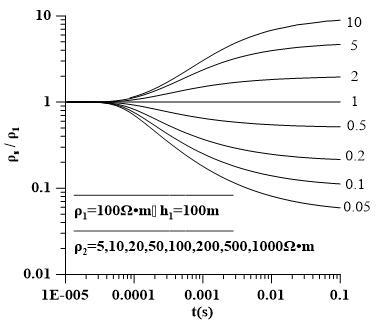Figure 6. Whole area apparent resistivity curve of the two-layered geo-electric model

5.2. Calculation of the whole area apparent resistivity of the three-layered geo-electric model

(1) Geo-electric model with variable second-layer resistivity. The three-layer geo-electric models can be divided into H-type, K-type, A-type and Q-type. Now we change the second-layer resistivity of the four typical three-layered geo-electric models, place the receiving points in different formation layers, and calculate the whole area apparent resistivity. Let the first-layer resistivity be $\rho_{1}$, the first-layer thickness $h_{1}$, the second-layer resistivity $\rho_{2}$, the second-layer thickness h2, and the third layer resistivity $\rho_{3}$. The model parameters are shown in Table 1.

Table 1. Parameters of the four geo-electric models with variable second-layer resistivity

 Model type ρ1 (Ω·m) h1 (m) ρ2 (Ω·m) h2 (m) ρ3 (Ω·m) H 100 100 90,70,50,20,10 100 100 K 100 100 200,500,1000,2000,5000 100 100 A 100 100 200,300,500,700,900 100 1000 Q 100 100 20,30,50,70,90 100 10

Figure 7~10 show the whole area apparent resistivity curves of the H-type, K-type, A-type and Q-type geo-electric models with the second-layer resistivity changing. As can be seen, for all the four geo-electric models, the whole area apparent resistivity curve can reflect the electrical structure of the corresponding formation. The initial section of the curve tends to the resistivity value of the first formation, and the tail section tends to the resistivity value of the third formation. The curve starts to deviate in the mid section, and as the difference increases between the second-layer resistivity value and those of the first layer and the third layer, the response amplitude of the whole area apparent resistivity curve becomes more significant. Among the four models, the H type and the K type show more significant response amplitudes.

## 7.jpg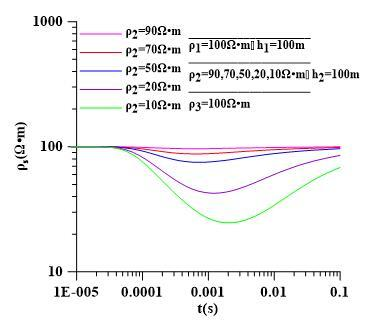Figure 7. Whole area apparent resistivity curve of the H type geo-electric model

## 8.jpg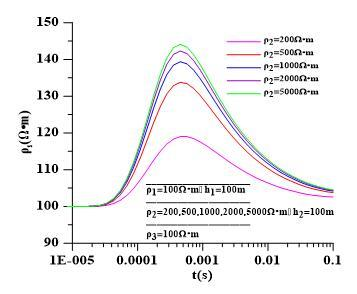Figure 8. Whole area apparent resistivity curve of the K type geo-electric model

## 9.jpg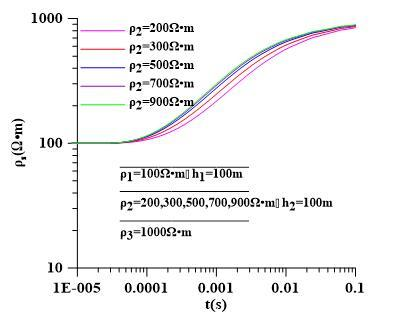Figure 9. Whole area apparent resistivity curve of the A type geo-electric model

## 10.jpgFigure 10. Whole area apparent resistivity curve of the Q type geo-electric model

(2) Geo-electric model with variable second-layer thickness. The parameters of the four typical three-layered geo-electric models with the second-layer thickness changing are shown in Table 2.

Table 2. Parameters of the three-layered geo-electric models with the second-layer thickness changing

 Model type ρ1 (Ω·m) h1 (m) ρ2 (Ω·m) h2 (m) ρ3 (Ω·m) H 100 100 10 20,50,80,120,150 100 K 10 100 100 20,50,80,120,150 10 A 10 100 50 20,50,80,120,150 100 Q 100 100 50 20,50,80,120,150 10

## 11.jpg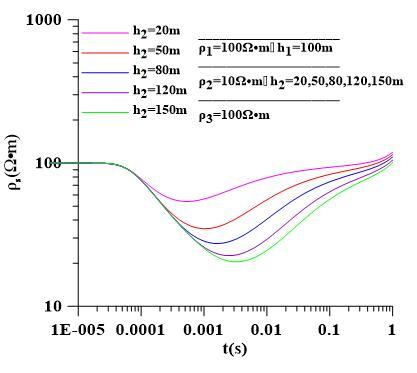Figure 11. Whole area apparent resistivity curve of the H type geo-electric model

## 12.jpg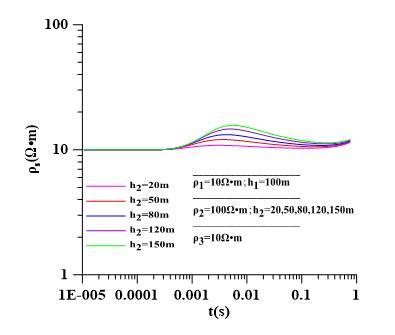Figure 12. Whole area apparent resistivity curve of the K type geo-electric model

## 13.jpg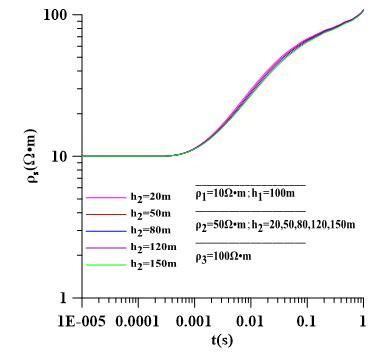Figure 13. Whole area apparent resistivity curve of the A type geo-electric model

## 14.jpgFigure 14. Whole area apparent resistivity curve of the Q type geo-electric model

Figure 11~14 show the whole area apparent resistivity curves of the H-type, K-type, A-type and Q-type geo-electric models with the second-layer thickness changing. As can be seen, the whole area apparent resistivity curve can well reflect the electrical features of the formation; with the thickness of the second layer increasing, the differences become more significant - the electrical response characteristics of the second layer are enhanced, and the response amplitude of the whole area apparent resistivity curve increases. Among the four models, the H-type sees the most obvious response amplitude of the whole area apparent resistivity curve.

(3) Geo-electric model with the first-layer, the second-layer and the third-layer resistivity and thicknesses changing. Considering the H-type whole area apparent resistivity curve has the most obvious response amplitude, this paper designs an H-type three-layered geo-electric model with the first-, second- and third-layer resistivity and thicknesses changing. The model parameters are shown in Table 3.

Figure 15~18 show the whole area apparent resistivity curves of the H-type and K-type geo-electric models with the first, second and third-layer resistivity and thicknesses changing, respectively. As can be seen, as the thickness of the first layer decreases, the initial section of the whole area apparent resistivity curve becomes more affected by the second-layer resistivity, the whole area apparent resistivity curve reflects the electrical information of the second layer earlier, and the response amplitude gradually increases; as the second-layer resistivity decreases, the response amplitude of the whole area apparent resistivity curve becomes larger; as the thickness of the second-layer increases, the response amplitude also becomes larger; and as the third-layer resistivity increases, the response amplitude also increases.

Table 3. Parameters of the three-layered geo-electric model with the first-, second- and third-layer resistivity and thicknesses changing

 Model type ρ1 (Ω·m) h1 (m) ρ2(Ω·m) h2 (m) ρ3 (Ω·m) H 100 20,50,80,120,150 10 100 100 H 100 100 5,10,20,50,80 100 100 H 100 100 10 100 100,200,500,800,1000 H 10 100 100 5,10,2050,100 100

## 15.jpg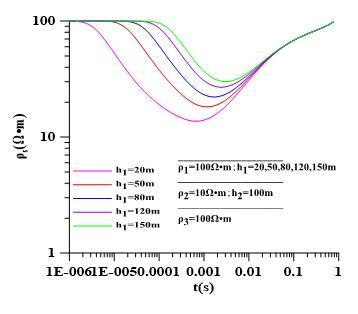Figure 15. Whole area apparent resistivity curve of the H-type model with the first-layer thickness changing

## 16.jpg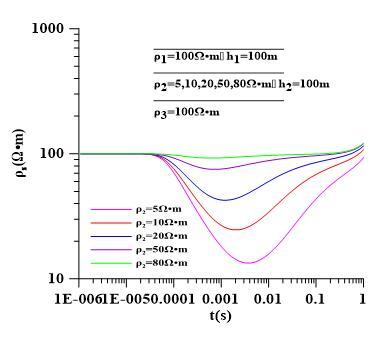Figure 16. Whole area apparent resistivity curve of the H-type model with the second-layer resistivity changing

## 17.jpg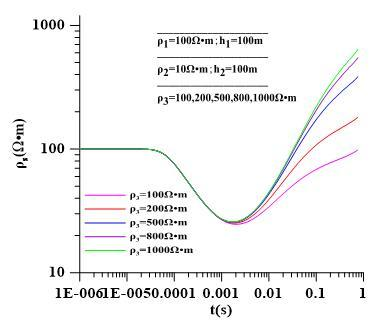Figure 17. Whole area apparent resistivity curve of the H-type model with the third-layer resistivity changing

## 18.jpg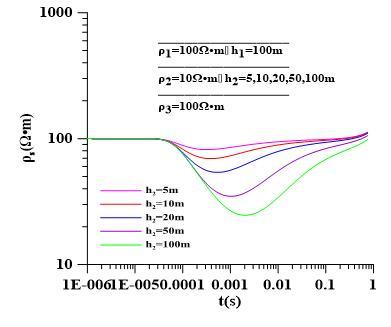Figure 18. Whole area apparent resistivity curve of the H-type model with the second-layer thickness changing

6. Conclusions

(1) The calculation results of the model show that the calculation method for the vertical magnetic field and the whole area apparent resistivity of the circular loop source over the layered medium is correct and effective;

(2) Through the calculation of different geo-electric models, it is found that the whole area apparent resistivity curve can well reflect the electrical features of the formation, and that the response magnitude of the H-type geo-electric model is the most significant;

(3) The whole area apparent resistivity curve has higher resolution for low resistance, and the lower the resistance, the greater the response amplitude and the larger the thickness of the layered medium.

(4) The circular loop device and the whole area apparent resistivity calculation method provide a solid theoretical basis for improving the mine TEM coils and the detection accuracy.

Acknoeledgments

“Thirteenth Five-Year Plan” the Key National R & D Program (On-line Dynamic Detection Technology and Equipment of Radio Wave Perspective in Mining Face 2018YFC0807805);

“Thirteenth Five-Year Plan” National Major Specially Funded Science and Technology Research Project (Downhole Cracking Effect Range Measurement Technology and Equipment 2016ZX05045004-007);

SAWS (State Administration of Work Safety) Funded Project (Census of Coal Mine Disaster Factors and Control Technology Equipment Research 2018-Technology and Equipment Department -Work Safety Research -01).

References

Amaya A. G., MRdh J., Dahlin T. (2018). Delimiting a saline water zone in quaternary fluvial–alluvial deposits using transient electromagnetic: A case study in Punata, Bolivia. Environmental Earth Sciences, Vol. 77, No. 2, pp. 46. https://doi.org/10.1007/s12665-017-7213-5

Anderson W. L. (2012). Computer program. numerical integration of related Hankel transforms of orders 0 and 1 by adaptive digital filtering. Geophysics, Vol. 44, No. 7, pp. 1287-1305. http://dx.doi.org/10.1190/1.1441007

Bai D. H., Meju M. A., Lu J., Wang L. F., He Z. H. (2003). Numerical calculation of apparent resistivity in time domain TEM central mode. Journal of Geophysics, Vol. 46, No. 5, pp. 697-704. http://dx.chinadoi.cn/10.3321/j.issn:0001-5733.2003.05.018

Chen M. S., Tian X. B. (1999). Study on transient electromagnetic sounding with electric couple source (V) - conversion of measured induced voltage into vertical magnetic field. Coalfield Geology and Exploration, No. 5, pp. 63-65. http://dx.chinadoi.cn/10.3969/j.issn.1001-1986.1999.05.018

Chen Q. L. (2009). Binary search algorithm for apparent resistivity of TEM in whole area. Journal of Petroleum and Natural Gas, Vol. 31, No. 2, pp. 45-49. http://dx.chinadoi.cn/10.3969/j.issn.1000-9752.2009.02.010

Christiansen A. V., Auken E., Kirkegaard C., Vignoli G. (2015). An efficient hybrid scheme for fast and accurate inversion of airborne transient electromagnetic data. Exploration Geophysics. http://dx.doi.org/10.1071/EG14121

Cui J. W., Xue G. Q., Chen W. Y., Deng J. Z. (2015). Calculation of apparent resistivity of electrical source transient electromagnetic field B. Progress in geophysics, Vol. 30, No. 6, pp. 2690-2697.

Fu Z. H., Sun T. C., Chen Q. L., Xie P. F. (2008). Numerical calculation of all-time apparent resistivity for ramp transient electromagnetic method. Journal of Electrical Technology, Vol. 23, No. 11, pp. 15-21. http://dx.chinadoi.cn/10.3321/j.issn:1000-6753.2008.11.003

Fullagar P. K., Pears G. A., Reid J. E., Schaa R. (2015). Rapid approximate inversion of airborne TEM. Exploration Geophysics, Vol. 46, No. 1, pp. 112. http://dx.doi.org/10.1071/EG14046

Guo S. W., Wang X. B. (2010). Study on numerical calculation method of apparent resistivity of TEM whole area. Geophysical and Geochemical Exploration Calculation Technology, Vol. 32, No. 5, pp. 500-507. http://dx.chinadoi.cn/10.3969/j.issn.1001-1749.2010.05.009

Guptasarma D., Singh B. (1997). New digital linear filters for Hankel J0 and J1 transforms. Geophysical Prospecting, Vol. 45, No. 5, pp. 745-762. http://dx.doi.org/10.1046/j.1365-2478.1997.500292.x

Li J. H., Zhu Z. Q., Liu S. C., Liu X. M., Zen S. H., Luo W. X. (2011). Transient electromagnetic fields excited by rectangular emission loops based on Gaver-Stehfest algorithm. Petroleum Geophysical Exploration, Vol. 46, No. 3, pp. 489-492.

Li J. P., Li T. L., Zhao X. F., Liang T. M. (2007). Study on the apparent resistivity of a layered medium with arbitrary loop source. Progress in Geophysics, No. 6, pp. 1777-1780. http://dx.chinadoi.cn/10.3969/j.issn.1004-2903.2007.06.015

Maclennan K., Li Y. (2011). Signal extraction from 4D transient electromagnetic surveys using the equivalent source method. Geophysics, Vol. 76, No. 3, pp. 47-52. http://dx.doi.org/10.1190/1.3599880

Schamper C., JRgensen F., Auken E., Efferso F. (2014). Assessment of near-surface mapping capabilities by airborne transient electromagnetic data — An extensive comparison to conventional borehole data. Geophysics, Vol. 79, No. 4, pp. B187-B199. http://dx.doi.org/10.1190/geo2013-0256.1

Wang H. J. (2008). Shifting algorithm of apparent resistivity in time domain transient electromagnetic method. Journal of Geophysics, Vol. 51, No. 6, pp. 1936-1942. http://dx.chinadoi.cn/10.3321/j.issn:0001-5733.2008.06.037

Wang T., Oristaglio M., Tripp A., Hohmann G. (1994). Inversion of diffusive transient elctromagnetic data by a conjugate-gradient method. Radio Science, Vol. 29, No. 4, pp. 1143-1156. http://dx.doi.org/10.1029/94RS00617

Yang H. Y., Deng J. Z., Zhang H., Yue J. H. (2010). Research on the interpretation method of all-space apparent resistivity of mine transient electromagnetic method. Journal of Geophysics, Vol. 53, No. 3, pp. 651-656. http://dx.chinadoi.cn/10.3969/j.issn.0001-5733.2010.03.020

Yu C. T., Liu H. F., Zhang X. J., Yang D. Y., Li Z. H. (2013). The analysis on IP signals in TEM response based on SVD. Applied Geophysics, Vol. 10, No. 1, pp. 79-87. https://doi.org/10.1007/s11770-013-0366-4